Llano, T., Arce, C., Ruiz, G., Chenna, N., and Coz, A. (2018). "Modelling and optimization of the last two stages of an environmentally-compatible TCF bleaching sequence," BioRes. 13(3), 6642-6662.

#### Abstract

A totally chlorine free (TCF) bleaching sequence was studied for an acid sulfite pulp mill that produces dissolving pulps. Laboratory analyses of the last two bleaching stages, an oxidant-reinforced alkaline extraction stage (EOP), and a subsequent pressurized peroxide with oxygen stage (PO), were performed on a eucalypt pulp that had been delignified by an ozone (Z) stage in the pulp mill. The goal was to predict the optimal costs and operational conditions for the (EOP)(PO) partial bleach sequence for three different specialty pulp products. Four independent variables affecting the pulp quality properties were examined for each stage (i.e., reaction temperature, reaction time, NaOH dosage and H2O2 dosage). The dependent variables were various pulp properties, such as intrinsic pulp viscosity, alpha-cellulose content, kappa number, and GE brightness. Three scenarios were considered to optimize the bleaching process, which related to a regenerated cellulose product (viscose) that is widely commercialized, and to two novel products (nanocrystalline cellulose (NCC) and nanofibrillated cellulose (NFC). Statistical response surface models indicated that the bleaching behavior of the ozone-treated pulp could be represented by second-order polynomial equations. These non-linear optimization models predict cost savings of 62.2%, 73.4%, and 63.3% for producing viscose, NCC, and NFC pulp grades, respectively.

Modelling and Optimization of the Last Two Stages of an Environmentally-Compatible TCF Bleaching Sequence

Tamara Llano,a Carlos Arce,a Gema Ruiz,a Naveen Chenna,b and Alberto Coz a,*

A totally chlorine free (TCF) bleaching sequence was studied for an acid sulfite pulp mill that produces dissolving pulps. Laboratory analyses of the last two bleaching stages, an oxidant-reinforced alkaline extraction stage (EOP), and a subsequent pressurized peroxide with oxygen stage (PO), were performed on a eucalypt pulp that had been delignified by an ozone (Z) stage in the pulp mill. The goal was to predict the optimal costs and operational conditions for the (EOP)(PO) partial bleach sequence for three different specialty pulp products. Four independent variables affecting the pulp quality properties were examined for each stage (i.e., reaction temperature, reaction time, NaOH dosage and H2O2 dosage). The dependent variables were various pulp properties, such as intrinsic pulp viscosity, alpha-cellulose content, kappa number, and GE brightness. Three scenarios were considered to optimize the bleaching process, which related to a regenerated cellulose product (viscose) that is widely commercialized, and to two novel products (nanocrystalline cellulose (NCC) and nanofibrillated cellulose (NFC). Statistical response surface models indicated that the bleaching behavior of the ozone-treated pulp could be represented by second-order polynomial equations. These non-linear optimization models predict cost savings of 62.2%, 73.4%, and 63.3% for producing viscose, NCC, and NFC pulp grades, respectively.

Keywords: Sulfite pulping; Dissolving pulp; Alkaline extraction; Peroxide bleaching; Regenerated cellulose

Contact information: a: Department of Chemistry and Process & Resources Engineering. Green Engineering and Resources Group, GER (www.geruc.es). University of Cantabria, 39005 Santander, Spain; b: Department of Bioproducts and Biosystems, School of Chemical Engineering, Aalto University. 00076 Aalto, Espoo, Finland; *Corresponding author: alberto.coz@unican.es

INTRODUCTION

Dissolving pulp is the raw material for the production of a wide range of cellulose derivatives, such as viscose staple fibers, cellulose esters, and ethers (Kumar and Christopher 2017). Dissolving pulp contains high levels of cellulose (90 to 98%) and exhibits very high brightness values (>90 % ISO) (Liu et al. 2016); consequently, these pulps have high-cellulose purity in comparison with mechanical, thermomechanical, semi-chemical, or some chemical pulps. Some processes that are being investigated to produce dissolving pulp are the SO2-ethanol-water (SEW) process (Iakovlev et al. 2011; Yahamoto et al. 2014) or the direct purification of paper-grade pulp (Roselli et al. 2014; Duan et al. 2017). Other well-known processes already manufacture dissolving pulps, such as pre-hydrolysis kraft (PHK) (Duan et al. 2015; Matin et al. 2015; Chen et al. 2016), pre-hydrolysis soda-anthraquinone (SAQ) (Sixta and Schild 2009) and acid sulfite (AS) (Llano et al. 2012; Llano et al. 2015).

Dissolving pulp demands have been growing worldwide during the last decade, especially in China. Sixta et al. (2013) described that the upturn of wood pulp in the market during the last 10 years might be attributed to a consistent growth for the regenerated cellulose fiber market. The recent outlook for the global dissolving pulp market published by RISI (2016) shows a demand increasing from 3.1 Mton/year in 2000 to 7.3 Mton/year in 2016; this outlook report estimates that this market will keep growing in subsequent years. This observation has evoked a huge interest in not only the pulping sector, but also in the textile and paper sectors; consequently, researchers worldwide are investigating this topic.

Bleaching is a crucial step in the manufacturing of dissolving pulps, whereby trace amounts of lignin, hemicelluloses, and other impurities are eliminated that are not removed during the chemical pulping process. More specifically, bleaching aims to increase pulp brightness and purity, while adjusting the cellulose’s viscosity and molecular weight distribution. Hence, bleaching accomplishes the following objectives: (i) to modify the reactivity of the cellulose in the resulting dissolving pulp; (ii) to meet customer requirements that utilize the dissolving pulp; and (iii) to increase brightness and purity of the dissolving pulp (Liu et al. 2016; Chen et al. 2016). There are numerous bleaching sequences employed to improve pulp reactivity and adjust pulp viscosity, such as chlorine-based bleaching (Gangwar et al. 2016), elemental chlorine free (ECF) (Bodhlyera et al. 2015; Li et al. 2015; Yao et al. 2016), catalytic bleaching (Kumar Chenna et al. 2016; Afsahi et al. 2018); total chlorine free (TCF) (Li et al. 2015; Zhou 2015; Veisi and Mahdavi 2016), and bio-bleaching with enzymes, such as cellulases (Duan et al. 2017; Hutterer et al. 2017), endoglucanase (Quintana et al. 2015a), xylanase (Hutterer et al.2017; Zhao et al. 2017), or laccase (García-Fuentevilla et al. 2015; Quintana et al. 2015b).

The research presented in this paper examines the last two stages of an industrial three-stage TCF bleach plant. The unbleached acid sulfite pulp enters the bleach plant and is further delignified by an ozone stage (Z); this stage was not considered in this study since the stage has a very short reaction time and the conditions of the stage is currently being optimized. The options studied following the Z stage was the oxidant-reinforced alkaline extraction (EOP), and the subsequent pressurized peroxide stage with oxygen (PO). These stages were investigated to see how they affected the physico-chemical properties of the resulting dissolving pulp prior to their conversion into viscose or nanocellulose pulp grades. In a previous publication (Llano et al. 2015), the entire Z(EOP)(PO) bleach sequence for the acid sulfite mill located in northern Spain was analyzed in general for viscose production. The authors concluded that the bleaching process could be improved to avoid molecular weight degradation of the cellulose. In this study, the (EOP) and (PO) stages will be evaluated to optimize the bleaching conditions to produce the three different dissolving pulp grade products. As was pointed out earlier, the scientific literature contains reports of chlorine-based, ECF, TCF or bio-bleaching sequences that accomplish different objectives. However, the literature does not address how to improve pulp quality when using TCF bleaching sequences to produce high alpha-cellulose dissolving pulp grades that are used for rayon (i.e., man-made regenerated fibers), or for nanocrystalline cellulose (NCC) and nanofibrillated cellulose (NFC).

The viscose was selected for study because of their large proportion in the global market. Liu et al. (2016) remarked that viscose (63% market share) is the most common product made from dissolving pulp.

Dissolving pulp grades that can be used for producing novel nanocelluloses, such as NFC and NCC, were also selected for study, as these are emerging new materials in the bio-based economy for pulp mills (García et al. 2016). Nanocellulose materials are becoming popular due to their feestocks and renewability; non-abrasive properties; high specific strength; and safer handling (Balea et al. 2016). This research will optimize the (EOP)(PO) partial bleach sequence in order to produce viscose grade as the mill currently produces, and also NFC and NCC grades. Hence, this will allow the acid sulfite pulp mill to diversify its business. Models developed from this work will permit the mill to use them as a predictive tool to assess the cost of producing cellulose derivatives without performing a comprehensive laboratory study.

Consequently, the objective of this research was to determine the optimal conditions for the (EOP)(PO) partial bleach sequence in relation to pulp grade specifications: alpha-cellulose content, intrinsic pulp viscosity, kappa number, and pulp brightness. In the first step of this study, a factorial design was performed. Then, mathematical modelling and optimizing of the (EOP)(PO) partial sequence was done by analyzing the effects of the main operational variables on pulp specifications. Bleaching optimization considered three scenarios for the production of different end-cellulose products: a regenerated cellulose product (rayon) and two novel nanocelluose products (CNF and NCC grades). Secondly, the empirical model was validated using a second set of experiment data, which tested intermediate operational conditions that were within the experimental space of the first step. Finally, techno-economic analyses were performed in order optimize the bleaching process to produce each of the three pulp grades with regards to economic parameters of chemical and energy costs. General Algebraic Modeling System (GAMS) software (GAMS Software GmbH, Frechen, Germany) was used to obtain the most cost-effective solution.

EXPERIMENTAL

Two sets of experiments were carried out for (EOP) and (PO) bleaching stages. First, a factorial experimental design was conducted that covered the combination of the four selected independent variables for each stage. Second, experiments were conducted that modified one of the four independent variables while keeping the remaining variables constant within the experimental space of the initial factorial design. Such is the aforementioned second set of experiments. They were useful for making the validation of the mathematical modelling. Conditions tested with the second round of experiments for the (EOP) stage were: 5, 10, 20, 30, 40, and 60 sodium hydroxide (NaOH) dosage expressed as kg/air-dried tonne (ADT); reaction times of 15, 30, 60, 90, 120 and 180 min; reaction temperatures of 80, 90, 120, 130 and 140 ºC; and hydrogen peroxide (H2O2) dosages of 0, 5, 10, 15, 20 and 40 kg/ADT. In the case of (PO) stage, the conditions tested were: 5, 10, 30, 40 and 60 NaOH dosage expressed as kg/ADT; reaction times of 30, 60, 90, 120, 150 and 180 min; reaction temperatures of 80, 100, 110, 120, 130 and 140 ºC; and H2O2 dosages of 5, 10, 20, 30, 40 and 60 kg/ADT.

Bleaching Procedure

Laboratory (EOP) and (PO) bleaching tests were conducted in 1 L stainless-steel reaction vessels. For the (EOP) stage, sodium hydroxide acts as the solubilizing agent for extracting modified lignin fragments together with oxygen and hydrogen peroxide, which further oxidizes and fragments the residual lignin. The (PO) stage uses hydrogen peroxide as the brightening agent in the presence of sodium hydroxide and oxygen.

The pulp used in these experiments was washed until resultant filtrate reached the pH of the tap water used to wash the pulp. Afterwards, the washed pulp was kept at 4 ºC to avoid carbohydrate degradation during storage (Yaqoob et al. 2010). Pulp (300 g) was mixed with H2O2 and NaOH with the amount of each reagent being dependent upon the statistical experimental design. Hot water (65 to 70 ºC) was added to achieve a pulp consistency value of 11%. The pulp was then introduced to the reactor, and the reactor was sealed and pressurized with oxygen at 1.65 bar for the (EOP) stage and 2.5 bar for the (PO) stage. The oxygen acting as delignifying agent was treated as a constant parameter and is not studied within this research. When the prescribed reaction time had expired, the reactor was depressurized and discharged; the resulting pulp was washed and laboratory handsheets were prepared prior to analyzing pulp properties.

Pulp Analysis

Pulp handsheets were prepared after pulp washing. Samples taken after (EOP) or (PO) treatment were disintegrated in a rotary stirrer to evenly disperse the pulp in water, filtered with a Büchner funnel and oven dried at 105 ºC. Afterwards, pulp handsheets were left to get moisture equilibrium in the air atmosphere.

Intrinsic pulp viscosity was measured in accordance to the ISO 5351 (2010) test standard. The oven-dried pulp (0.2500±0.0005 g) was dispersed in 25 mL of distilled/ deionized water, 25 mL 1.0 M curpiethylendiamine solution mixed with the dispersion, and the pulp was mixed until it was fully dissolved. The resulting solution was placed into a Cannon-Fenske 150 viscometer, which was placed into a 25 ºC water bath. The efflux time of the solution to pass through the viscometer was measured. The intrinsic pulp viscosity value was calculated according to the test method procedure.

The amount of alpha-cellulose was determined in accordance to TAPPI T203 cm-99 (1999). An oven-dried pulp sample (0.5±0.1 g) was extracted with a 17.5% NaOH solution at 25±0.2 ºC. A 25 mL portion of the resulting filtrate was taken and mixed with 10 mL of 0.5 N K2Cr2O7 and 50 mL of 96 % H2SO4. After 15 min, samples were cooled by adding 50 mL of water, which was then titrated with 0.1 N (NH4)2Fe(SO4)2·6H2O using ferroin as the end-point indicator.

Kappa number test estimates the amount of residual lignin and hexenuronic acid by measuring the oxidant demand of the pulp. TAPPI Useful Method UM 246 (1991) was used to estimate the kappa number. TAPPI Useful Method UM 246 is known as the micro kappa number test and is used for high-purity pulps, such as partially and fully bleached pulps with high cellulose content and kappa numbers below 5.

Brightness represents the numerical value of the reflectance factor of a sample with respect to blue light of specific spectral and geometric characteristics. The method is described in the TAPPI Test Method T452 om-08 (2008), and is known as GE (or TAPPI) brightness. The equipment required consist of a reflectometer employing 45° illumination and 0° viewing geometry (45°/0°) using light with an effective wavelength of 457±0.5 nm.

Experimental Design

The independent variables of reaction time, reaction temperature, NaOH dosage, and H2O2 dosage for each stage were modulated in accordance to a statistically-designed three-level, four-factor factorial experimental design. The independent variables were evaluated at three levels: low (-1), medium (0) and high (+1). Ranges of values for the independent variables used in the experimental design at each stage of the (EOP)(PO) partial sequence are shown in Table 1. The independent variables were normalized (or converted to coded units) from -1 to +1 in order to facilitate direct comparison of the coefficients and visualization of the effects of the individual independent variables on the response variable (Eq. 1),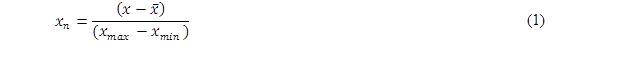where xn is the coded value of independent variable nx is the variable’s uncoded value, is the average value of the variable, xmax is the maximum value of the variable, and xmin is the minimum value of the variable. Normalization of the independent variables reduces interrelationships between linear and quadratic terms in the developed response surface model (Montgomery 1997).

Experimental errors were calculated from the central points of the statistical experimental design (i.e., first set of experiments) for the (EOP) and (PO) stages. In all cases, the experimental errors were lower than: 10% for the kappa number; 1% for the alpha-cellulose content; 5% for the GE brightness; and 5% for the intrinsic pulp viscosity.

Table 1. Ranges of the Independent Variables Used in the Experimental Design at (EOP) and (PO) Stages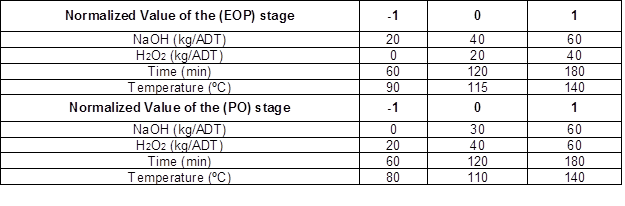The total number of experiments carried out for each stage in the statistical design are illustrated in Fig. 1. The number of experiments ran are calculated by considering: the number of variables (k), which is equal to 4; a constant p that is equal to zero at k<5 and to 1 at k>5; and the number of central points (nc), which is equal to 1. Hence, the number of experiments for 2k-p factor design points, 2∙k star points and nc central points is calculated as (Eq. 2):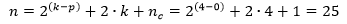(2)

There are 16 factor design experiments, eight star point experiments and one central point, which yielded a total of 25 experiments for each stage (50 experiments total).

Apart from the above experimental design, additional laboratory bleaching experiments of (EOP) and (PO) stages were conducted by modifying one of the independent variables while keeping the remaining variables constant. Such is the aforementioned second set of experiments. These experiments were used to validate the second-order response surface model derived from the earlier statistical design-of-experiments for each bleaching stage.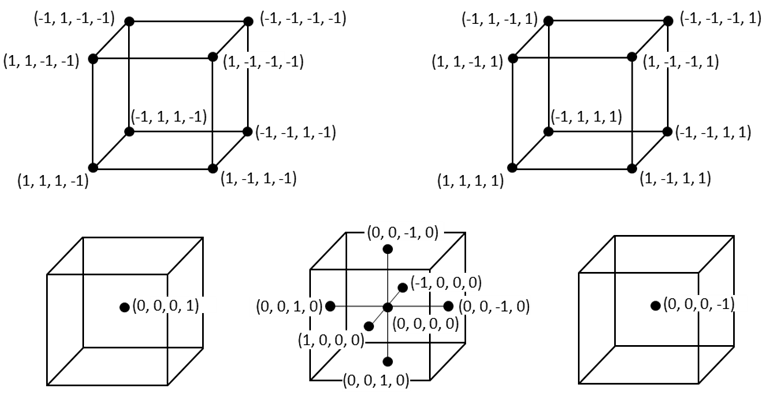Fig. 1. Plot of the coordinates for the experiments included in the experimental design that are based on four independent variables for each bleaching stage

ANOVA analysis was done to obtain the coefficients that were included in the GAMS software and to obtain statistics such as R2, standard error, and F-value. Pareto charts were constructed to graphically determine the most important effects with a statistical confidence level of 95% and their impact over the quality pulp parameters. Response surface graphs were plotted since they present useful information about the behavior of a response variable in the whole studied range. Additionally, the Parity plots reveal how mathematical models are useful in the factorial experimental design and also in other conditions inside the system boundaries.

Mathematical Modelling

The statistical design-of-experiments enabled the construction of the second-order response surface model, and the detection of statistical significance with the variables examined. The dependent variable responses were predicted using the second-order response surface model of the form,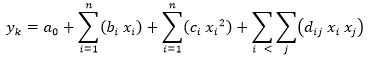(3)

where xi are the independent variables (uncoded values); ykdependent variables; n the number of independent variables (n=4); and k is the number of dependent variables (k=4). The terms bici and dij denote coefficient terms for linear, quadratic, and binary interaction terms of the independent variables; the a0 term denotes the intercept value for dependent variable yk. All terms of the models with statistical p-values higher than 0.05 were not included, which represented a statistical confidence level of 95%. The coefficients and factors for the response surface models were determined from the analysis of the experimental data using Statgraphics statistical software (Statpoint Technologies, Inc, The Plains, VA, USA).

Optimization

The mathematical model developed from the statistical design-of-experiments was used for the optimization of the bleaching process with the GAMS software. Optimization considered the key pulp specifications. Not all end-cellulose products have the same pulp specifications; hence, three different models were developed for optimum bleaching for producing: (i) viscose; (ii) nanocrystalline cellulose; and (iii) nanofibrillated cellulose grades.The general objective function (Z) for the three scenarios is the same and consists of minimizing the expression (Eq. 4):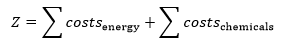(4)

Costs related to energy (costsenergy) and chemical (costschemical) consumption for each stage were considered since they are the major contributors to bleaching costs. Energy costs for (EOP) and (PO) stages were calculated by Eqs. 5 and 6, respectively: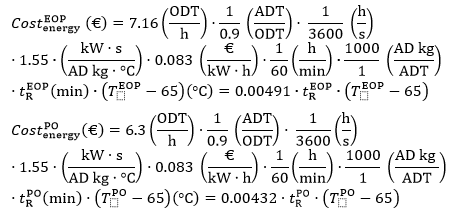Individual chemical costs for the (EOP) stage ((Eqs. 7, 8, and 9) and the (PO) stage (Eqs. 10, 11 and 12) were calculated. For the chemical costs they were considered consumptions of NaOH, H2O2 (process variables studied in this work) and oxygen (considered as a constant in this work) but also consumed during the bleaching sequence.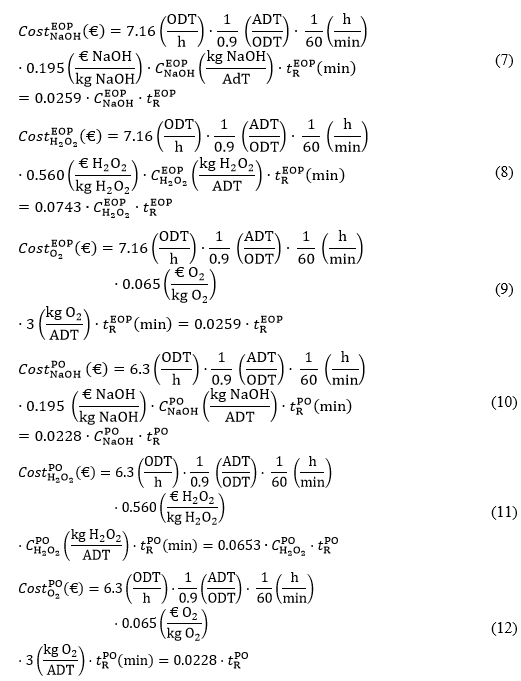Equations 4 to 12 were developed by taking into account data provided by the pulp mill, and considering the mass balances, reagent prices, production, and consumption per Air Dried Tonne (ADT) of dissolving pulp. With regard to the energy cost of 0.083 €/KW·h, this data was taken from the Ministry of Commerce Industry and Tourism (MINCOTUR 2018). The only costs considered in the three optimization models were the major contributors to the bleaching costs, which correspond to reagent and energy costs. Water consumption or labour costs were not introduced in the models.

Pulp production rates of oven-dried tonne (ODT) basis were converted to air-dried tonne (ADT) basis assuming a 10% moisture content. Costs equations (Eqs. 5 to 12) were expressed as functions of the independent variables of , , and , which were bleach stage reaction times, sodium hydroxide and peroxide dosages, and reaction temperatures for (PO) and (EOP), respectively. Apart from the costs, equations of the response variables (kappa number, alpha-cellulose content, intrinsic pulp viscosity and pulp brightness) as a function of the operational variables (reaction time, peroxide dosage, sodium hydroxide dosage and reaction temperature) were also introduced in the GAMS software. These equations are presented in the Results and Discussion section (Table 3). The whole system of equations constitutes a non-linear problem (NLP) for optimization.

Bleaching chemical pulps to high brightness requires a multi-stage bleach sequence. The first stage or two of the sequence focuses on removing residual lignin in the pulp and are known as delignification stages. The subsequent stages in the sequence are the brightening stages in which the chromophores in the pulp are eliminated (Sixta et al. 2006). In this study, the optimization of Z(EOP)(PO) focused on the (EOP)(PO) partial sequence, where the models for the (EOP) and (PO) stages were considered together when optimizing for the three different scenarios.

Once the objective function was defined and established with the rest of equations, some restrictions were incorporated to refine the objective function. Such restrictions vary as a function of the pulp grade and are summarized in Table 2. These restrictions should be accomplished at the end of the (EOP)(PO) partial sequence in order to meet the product specifications of the dissolving pulp grade.

Constraints applied to the (EOP) stage are the same for all dissolving pulp grades. (EOP) restrictions were: 89 to 90% alpha-cellulose content, 70 to 80% brightness (GE), intrinsic pulp viscosity of 550 to 610 mL/g, and kappa number of 1.0 to 2.0. In the case of the (PO) stage, the product specifications for viscose, NCC and NFC pulp grades were taken from the literature (Abraham et al. 2011; Sehaqui et al. 2011; Tonoli et al. 2012; Wang et al. 2012; Zhao et al. 2013; Barbash et al. 2017a; Barbash et al. 2017b). These (PO) stage constraints for each pulp grade are shown in Table 2.

Table 2. Final Quality Pulp Properties for the Models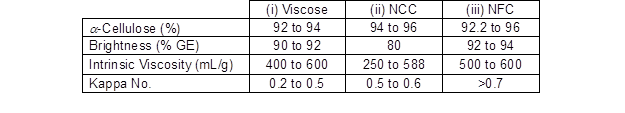RESULTS AND DISCUSSION

Raw Material Characterization

Pulp samples provided by the Sniace S.A. pulp mill (Torrelavega, Spain) were characterized prior to conducting the bleaching experiments. The acid sulfite pulp was made from Eucalyptus globulus. The pulp used for laboratory (EOP) bleaching had undergone an ozone delignification (Z) stage at the mill, and had a kappa number of 2.89±0.21, an alpha-cellulose content of 88.9±0.3%, an intrinsic pulp viscosity of 625±30 mL/g, and a GE brightness of 66.8±2.5%. The pulp used for laboratory (PO) bleaching had been obtained after the mill’s Z(EOP) partial bleach sequence, and had a kappa number of 1.76±0.11, alpha-cellulose of 90.9±0.8%, intrinsic viscosity of 557±26.2 mL/g, and a GE brightness of 69.8±2.0%.

Experimental Design and Mathematical Modelling

The first set of experiments (Tables 1 and 2) were used to perform a mathematical model capable to predict the response variables as a function of the four independent selected variables within the range of study. Second-order response surface models obtained from the design-of-experiments fitted the data well; the regression coefficients of the statistical surface models and F-values are provided in Table 3. The coefficients of the mathematical adjustment are shown in Table 3. The equation is given by Eq.13.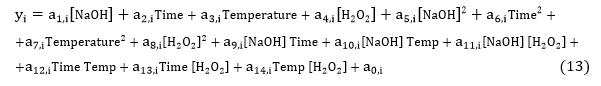Statistical analyses revealed how well the data fitted with second-order polynomial. These empirical models describe end-quality pulp properties as a function of the operational variables of the (EOP) and (PO) stages (i.e., reaction time, reaction temperature, NaOH dosage, and H2O2 dosage). Only significant factors giving a p-value less than 0.05 were considered in the final response surface models. Pareto charts (Fig. 3) indicated that the most influential variables affecting the end-quality pulp characteristics. The standardized effect (positive or negative) for each independent variable is also represented in the Pareto charts. Three-dimensional graphs of each dependent variable against the two most influential variables are displayed in Fig. 4 for (EOP) and (PO) stages.

Table 3. Coefficients for Each Independent Variable in the Models to Predict Dependent Variables for (EOP) and (PO) Stages with ANOVA Analyses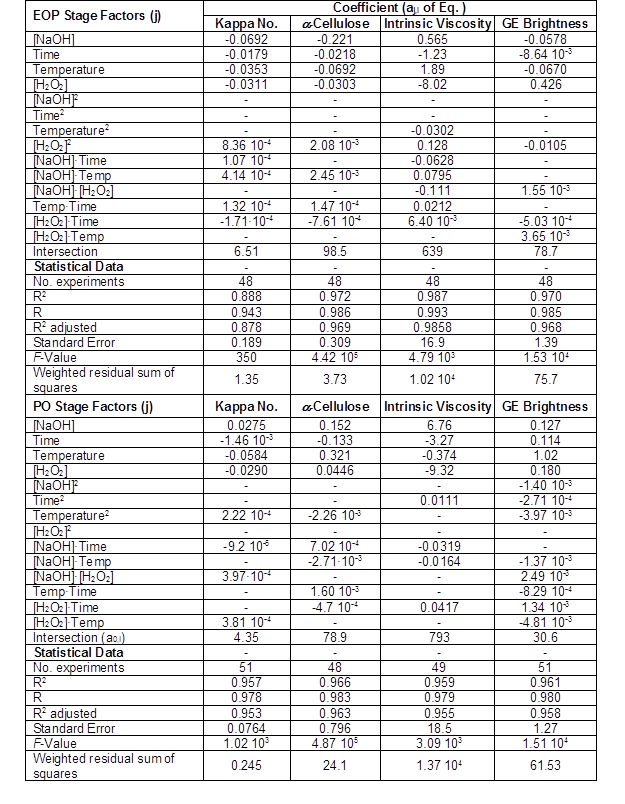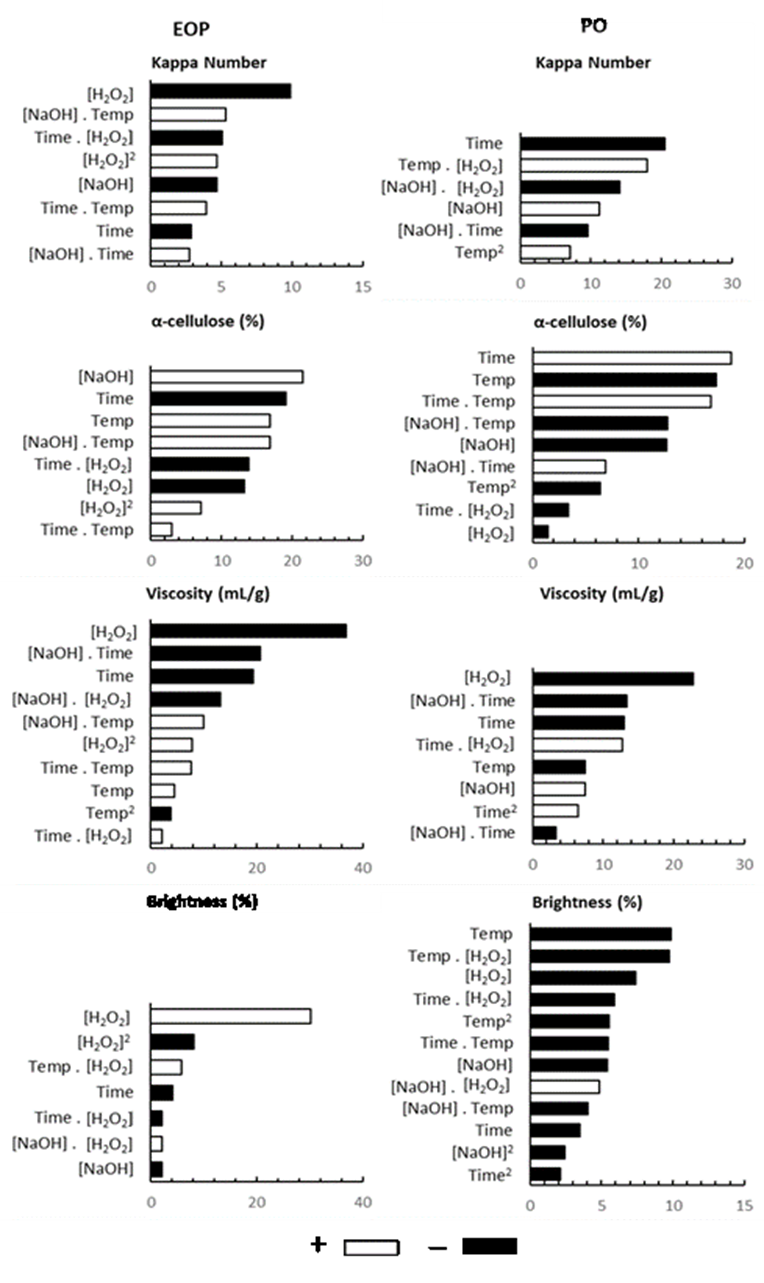Fig. 3. Pareto charts of standardized effects for (EOP) and (PO) stages